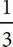# SAT Math Multiple Choice Question 207: Answer and Explanation

### Test Information

Question: 207

12. x + 3y = 42
3x - y = 8

In the system of equations above, how many points of intersection do the equations share and what is their relationship, if any?

• A. Zero, and the lines are parallel.
• B. Infinitely many, and the lines are the same line.
• C. One, and the lines have no relationship.
• D. One, and the lines are perpendicular.

D First, rewrite the equations so that they are in the slope-intercept form of a line, y = mx + b, where m = slope. The first equation becomes 3y = -x + 42 or y = -x + 14. The slope of this first line is therefore -. The second equation becomes -y = -3x + 8 or y = 3x - 8. The slope of this line is therefore 3. The slopes of the two lines are negative reciprocals of each other, which means that the two lines are perpendicular to each other. The correct answer is (D).